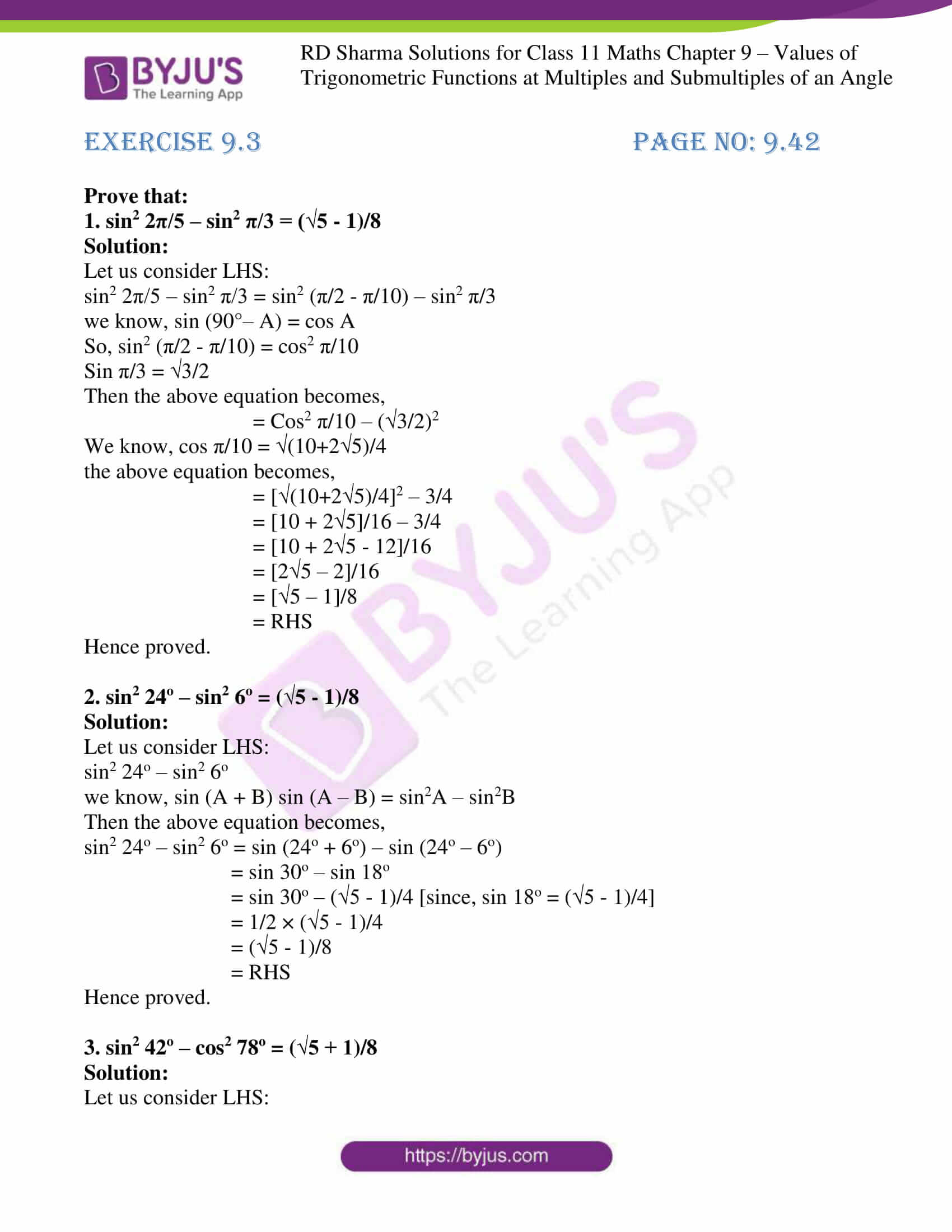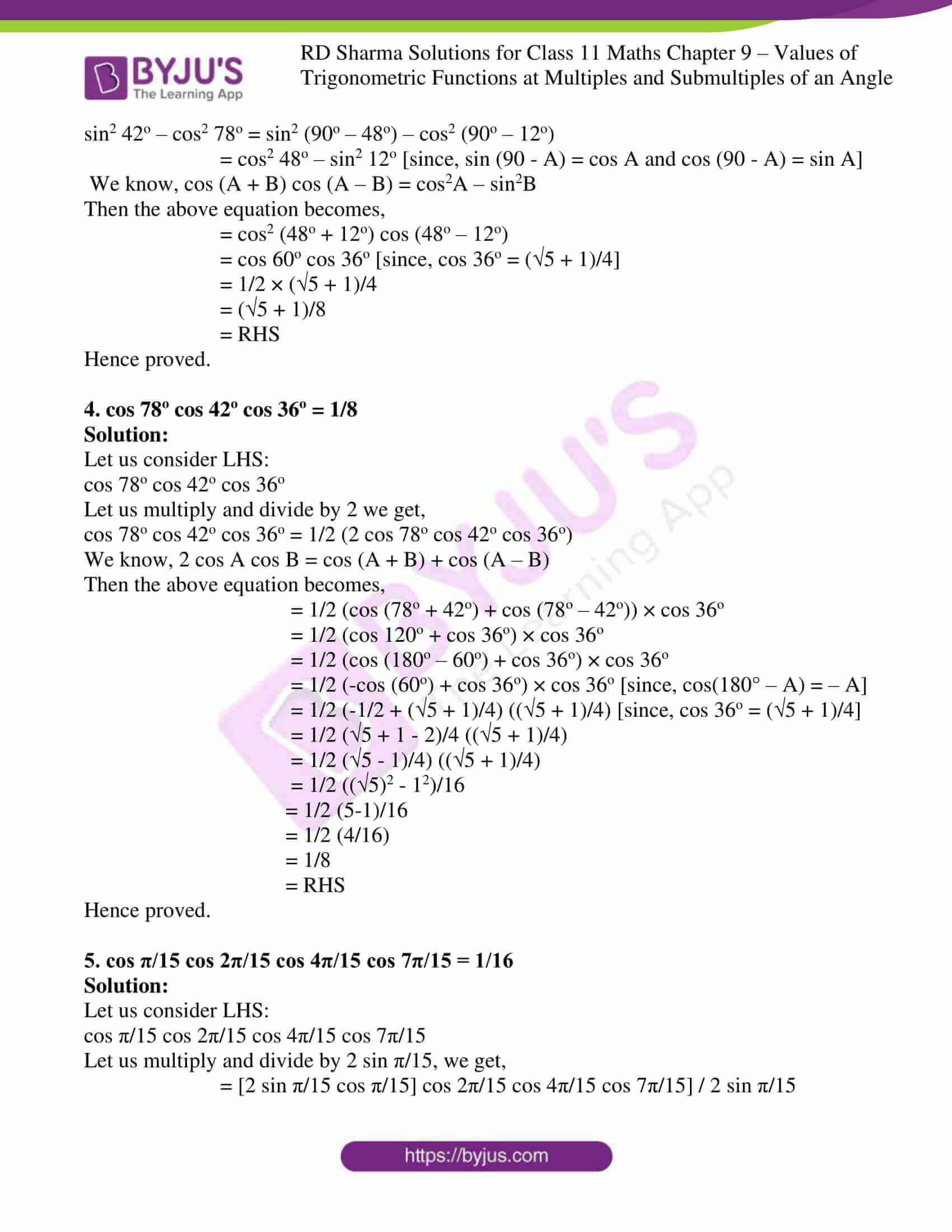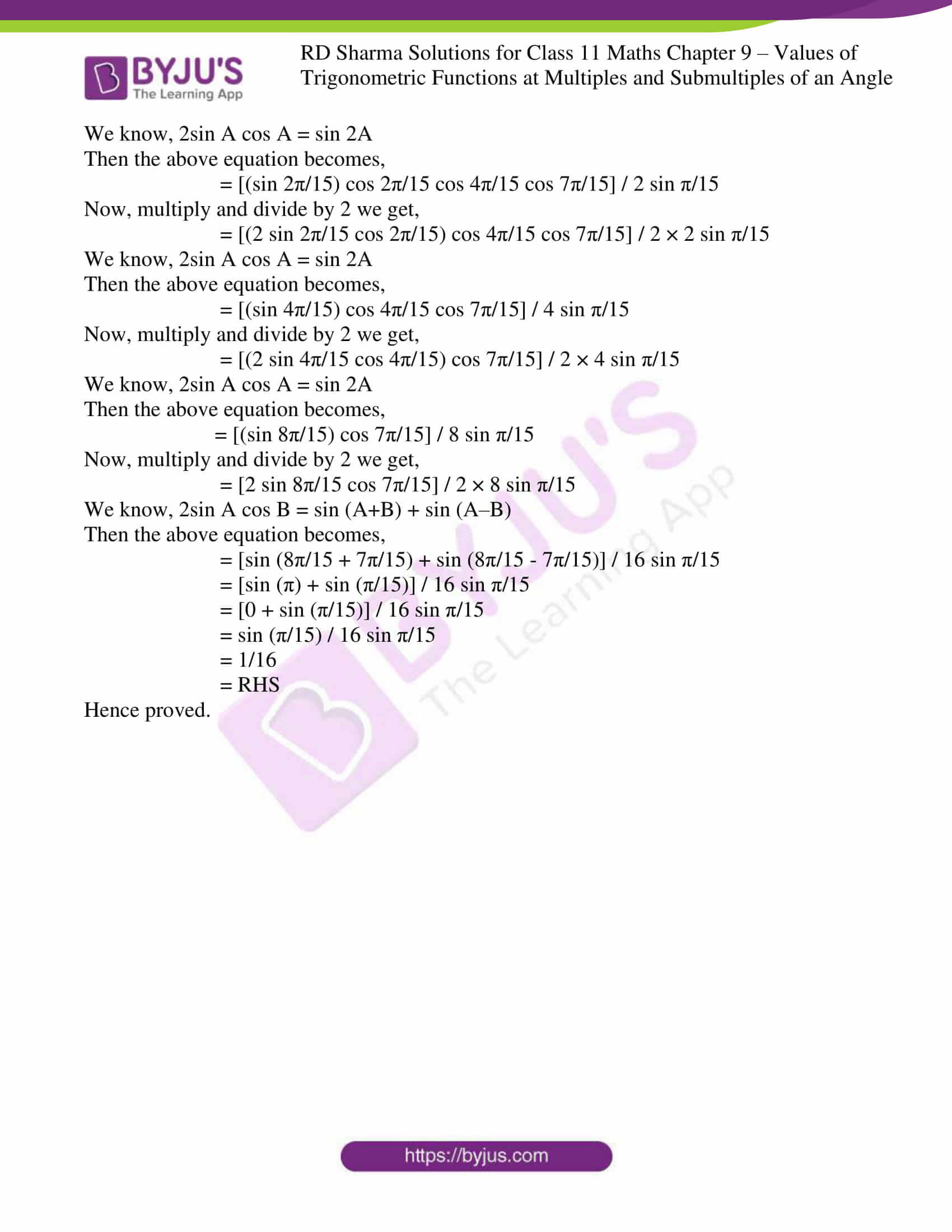This Exercise deals with problems based on the values of trigonometric functions at ‘x’ in terms of values at ‘x/3’. The solutions here are explained in the most simple and understandable language which helps students to improve their grasping abilities. The presentation of each solution in this exercise is described in a unique way by the expert tutors at BYJU’S. To score good marks, practising RD Sharma Class 11 Maths Solutions can help to a great extent. The pdf of RD Sharma Solutions for Class 11 Maths Chapter 9 exercise 9.3 is provided here, which students can download for free easily.

## Download the Pdf of RD Sharma Solutions for Class 11 Maths Exercise 9.3 Chapter 9 – Values of Trigonometric Functions at Multiples and Submultiples of an Angle### Also, access other exercises of RD Sharma Solutions for Class 11 Maths Chapter 9 – Values of Trigonometric Functions at Multiples and Submultiples of an Angle

Exercise 9.1 Solutions

Exercise 9.2 solutions

### Access answers to RD Sharma Solutions for Class 11 Maths Exercise 9.3 Chapter 9 – Values of Trigonometric Functions at Multiples and Submultiples of an Angle

Prove that:

1. sin2 2π/5 – sin2 π/3 = (√5 – 1)/8

Solution:

Let us consider LHS:

sin2 2π/5 – sin2 π/3 = sin2 (π/2 – π/10) – sin2 π/3

we know, sin (90°– A) = cos A

So, sin2 (π/2 – π/10) = cos2 π/10

Sin π/3 = √3/2

Then the above equation becomes,

= Cos2 π/10 – (√3/2)2

We know, cos π/10 = √(10+2√5)/4

the above equation becomes,

= [√(10+2√5)/4]2 – 3/4

= [10 + 2√5]/16 – 3/4

= [10 + 2√5 – 12]/16

= [2√5 – 2]/16

= [√5 – 1]/8

= RHS

Hence proved.

2. sin2 24o – sin2 6o = (√5 – 1)/8

Solution:

Let us consider LHS:

sin2 24o – sin2 6o

we know, sin (A + B) sin (A – B) = sin2A – sin2B

Then the above equation becomes,

sin2 24o – sin2 6o = sin (24o + 6o) – sin (24o – 6o)

= sin 30o – sin 18o

= sin 30o – (√5 – 1)/4 [since, sin 18o = (√5 – 1)/4]

= 1/2 × (√5 – 1)/4

= (√5 – 1)/8

= RHS

Hence proved.

3. sin2 42o – cos2 78o = (√5 + 1)/8

Solution:

Let us consider LHS:

sin2 42o – cos2 78o = sin2 (90o – 48o) – cos2 (90o – 12o)

= cos2 48o – sin2 12o [since, sin (90 – A) = cos A and cos (90 – A) = sin A]

We know, cos (A + B) cos (A – B) = cos2A – sin2B

Then the above equation becomes,

= cos2 (48o + 12o) cos (48o – 12o)

= cos 60o cos 36o [since, cos 36o = (√5 + 1)/4]

= 1/2 × (√5 + 1)/4

= (√5 + 1)/8

= RHS

Hence proved.

4. cos 78o cos 42o cos 36o = 1/8

Solution:

Let us consider LHS:

cos 78o cos 42o cos 36o

Let us multiply and divide by 2 we get,

cos 78o cos 42o cos 36o = 1/2 (2 cos 78o cos 42o cos 36o)

We know, 2 cos A cos B = cos (A + B) + cos (A – B)

Then the above equation becomes,

= 1/2 (cos (78o + 42o) + cos (78o – 42o)) × cos 36o

= 1/2 (cos 120o + cos 36o) × cos 36o

= 1/2 (cos (180o – 60o) + cos 36o) × cos 36o

= 1/2 (-cos (60o) + cos 36o) × cos 36o [since, cos(180° – A) = – A]

= 1/2 (-1/2 + (√5 + 1)/4) ((√5 + 1)/4) [since, cos 36o = (√5 + 1)/4]

= 1/2 (√5 + 1 – 2)/4 ((√5 + 1)/4)

= 1/2 (√5 – 1)/4) ((√5 + 1)/4)

= 1/2 ((√5)2 – 12)/16

= 1/2 (5-1)/16

= 1/2 (4/16)

= 1/8

= RHS

Hence proved.

5. cos π/15 cos 2π/15 cos 4π/15 cos 7π/15 = 1/16

Solution:

Let us consider LHS:

cos π/15 cos 2π/15 cos 4π/15 cos 7π/15

Let us multiply and divide by 2 sin π/15, we get,

= [2 sin π/15 cos π/15] cos 2π/15 cos 4π/15 cos 7π/15] / 2 sin π/15

We know, 2sin A cos A = sin 2A

Then the above equation becomes,

= [(sin 2π/15) cos 2π/15 cos 4π/15 cos 7π/15] / 2 sin π/15

Now, multiply and divide by 2 we get,

= [(2 sin 2π/15 cos 2π/15) cos 4π/15 cos 7π/15] / 2 × 2 sin π/15

We know, 2sin A cos A = sin 2A

Then the above equation becomes,

= [(sin 4π/15) cos 4π/15 cos 7π/15] / 4 sin π/15

Now, multiply and divide by 2 we get,

= [(2 sin 4π/15 cos 4π/15) cos 7π/15] / 2 × 4 sin π/15

We know, 2sin A cos A = sin 2A

Then the above equation becomes,

= [(sin 8π/15) cos 7π/15] / 8 sin π/15

Now, multiply and divide by 2 we get,

= [2 sin 8π/15 cos 7π/15] / 2 × 8 sin π/15

We know, 2sin A cos B = sin (A+B) + sin (A–B)

Then the above equation becomes,

= [sin (8π/15 + 7π/15) + sin (8π/15 – 7π/15)] / 16 sin π/15

= [sin (π) + sin (π/15)] / 16 sin π/15

= [0 + sin (π/15)] / 16 sin π/15

= sin (π/15) / 16 sin π/15

= 1/16

= RHS

Hence proved.